# Excel COUNTIF and COUNTIFS with OR conditions

The tutorial explains how to use Excel's COUNTIF and COUNTIFS functions to count cells with multiple OR as well as AND conditions.

As everyone knows, Excel COUNTIF function is designed to count cells based on just one criterion while COUNTIFS evaluates multiple criteria with AND logic. But what if your task requires OR logic - when several conditions are provided, any one can match to be included in the count?

There are a few possible solutions to this task, and this tutorial will cover them all in full detail. The examples imply that you have a sound knowledge of the syntax and general uses of both functions. If not, you may want to begin with revising the basics:

Excel COUNTIF function - counts cells with one criteria.

Excel COUNTIFS function - counts cells with multiple AND criteria.

Now that everyone is on the same page, let's dive in:

## Count cells with OR conditions in Excel

This section covers the simplest scenario - counting cells that meet any (at least one) of the specified conditions.

### Formula 1. COUNTIF + COUNTIF

The easiest way to count cells that have one value or another (Countif a or b) is to write a regular COUNTIF formula to count each item individually, and then add the results:

COUNTIF(range, criterion1) + COUNTIF(range, criterion2)

As an example, let's find out how many cells in column A contain either "apples" or "bananas":

`=COUNTIF(A:A, "apples") + COUNTIF(A:A, "bananas")`

In real-life worksheets, it is a good practice to operate on ranges rather than entire columns for the formula to work faster. To spare the trouble of updating your formula every time the conditions change, type the items of interest in predefined cells, say F1 and G1, and reference those cells. For example:

`=COUNTIF(A2:A10, F1) + COUNTIF(A2:A10, G1)`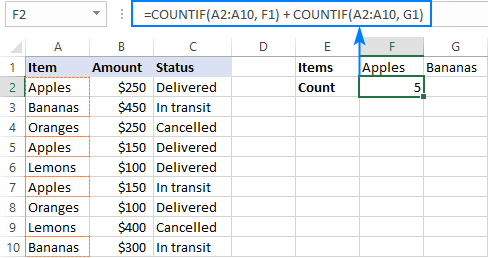This technique works fine for a couple of criteria, but adding three or more COUNTIF functions together would make the formula too cumbersome. In this case, you'd better stick with one of the following alternatives.

### Formula 2. COUNTIF with array constant

Here's a more compact version of the SUMIF with OR conditions formula in Excel:

SUM(COUNTIF(range, {criterion1, criterion2, criterion3, …}))

The formula is constructed in this way:

First, you package all the conditions in an array constant - individual items separated by commas and the array enclosed in curly braces like {"apples", "bananas', "lemons"}.

Then, you include the array constant in the criteria argument of a normal COUNTIF formula: COUNTIF(A2:A10, {"apples","bananas","lemons"})

Finally, warp the COUNTIF formula in the SUM function. It is necessary because COUNTIF will return 3 individual counts for "apples", "bananas" and "lemons", and you need to add those counts together.

Our complete formula goes as follows:

`=SUM(COUNTIF(A2:A10,{"apples","bananas","lemons"}))`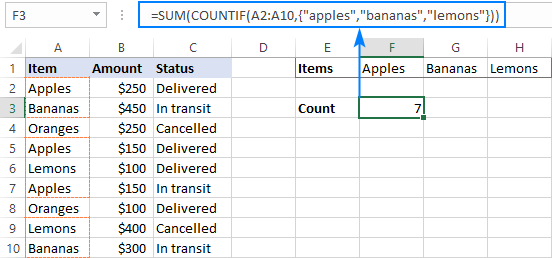If you'd rather supply your criteria as range references, you'll need to enter the formula with Ctrl + Shift + Enter to make it an array formula. For example:

`=SUM(COUNTIF(A2:A10,F1:H1))`

Please notice the curly braces in the screenshot below - it is the most evident indication of an array formula in Excel: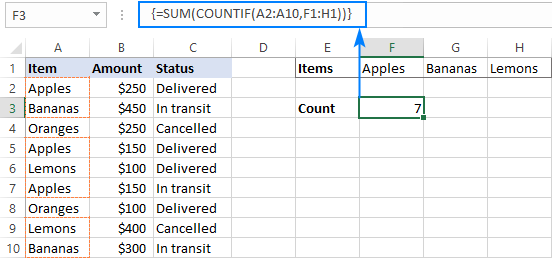### Formula 3. SUMPRODUCT

Another way to count cells with OR logic in Excel is to use the SUMPRODUCT function in this way:

SUMPRODUCT(1*(range ={criterion1, criterion2, criterion3, …}))

To better visualize the logic, this could also be written as:

SUMPRODUCT((range=criterion1) + (range=criterion2) + …)

The formula tests each cell in the range against each criterion and returns TRUE if the criterion is met, FALSE otherwise. As an intermediate result, you get a few arrays of TRUE and FALSE values (the number of arrays equals the number of your criteria). Then, the array elements in the same position are added together, i.e. the first elements in all the arrays, the second elements, and so on. The addition operation converts the logical values to numbers, so you end up with one array of 1's (one of the criteria matches) and 0's (none of the criteria matches). Because all the criteria are tested against the same cells, there is no way any other number could appear in the resulting array - only one initial array can have TRUE in a specific position, others will have FALSE. Finally, SUMPRODUCT adds up the elements of the resulting array, and you get the desired count.

The first formula works in a similar manner, with the difference that it returns one 2-dimentional array of TRUE and FALSE values, which you multiply by 1 to convert the logical values to 1 and 0, respectively.

Applied to our sample data set, the formulas take the following shape:

`=SUMPRODUCT(1*(A2:A10={"apples","bananas","lemons"}))`

Or

`=SUMPRODUCT((A2:A10="apples") + (A2:A10="bananas") + (A2:A10="lemons"))`

Replace the hardcoded array constant with a range reference, and you will get even a more elegant solution:

`=SUMPRODUCT(1*( A2:A10=F1:H1))`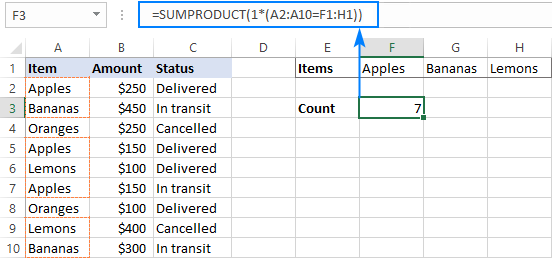Note. The SUMPRODUCT function is slower than COUNTIF, which is why this formula is best to be used on relatively small data sets.

## Count cells with OR as well as AND logic

When working with large data sets that have multi-level and cross-level relations between elements, chances are that you will need to count cells with OR and AND conditions at a time.

As an example, let's get a count of "apples", "bananas" and "lemons" that are "delivered". How do we do that? For starters, let's translate our conditions into Excel's language:

• Column A: "apples" or "bananas" or "lemons"
• Column C: "delivered"

Looking from another angle, we need to count rows with "apples and delivered" OR "bananas and delivered" OR "lemons and delivered". Put this way, the task boils down to counting cells with 3 OR conditions - exactly what we did in the previous section! The only difference is that you'll utilize COUNTIFS instead of COUNTIF to evaluate the AND criterion within each OR condition.

### Formula 1. COUNTIFS + COUNTIFS

It is the longest formula, which is the easiest to write :)

`=COUNTIFS(A2:A10, "apples", C2:C10, "delivered") + COUNTIFS(A2:A10, "bananas", C2:C10, "delivered")) + COUNTIFS(A2:A10, "lemons", C2:C10, "delivered"))`

The screenshot below shows the same formula with cells references:

`=COUNTIFS(A2:A10, K1, C2:C10, K2) + COUNTIFS(A2:A10, L1, C2:C10, K2) + COUNTIFS(A2:A10, M1,C2:C10, K2)`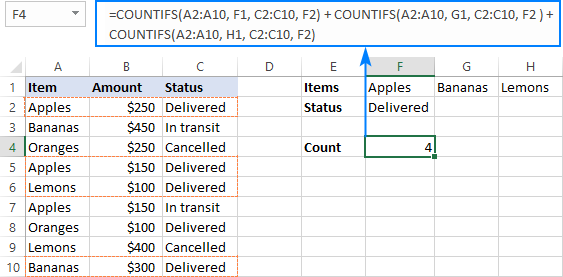### Formula 2. COUNTIFS with array constant

A more compact COUNTIFS formula with AND/OR logic can be created by packaging OR criteria in an array constant:

`=SUM(COUNTIFS(A2:A10, {"apples","bananas","lemons"}, C2:C10, "delivered"))`

When using a range reference for the criteria, you need an array formula, completed by pressing Ctrl + Shift + Enter:

`=SUM(COUNTIFS(A2:A10,F1:H1,C2:C10,F2))`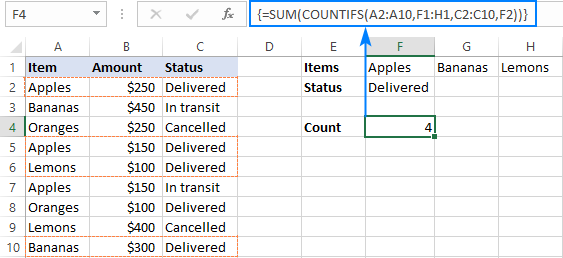Tip. If needed, you are free to use wildcards in the criteria of any formulas discussed above. For example, to count all sorts of bananas such as "green bananas" or "goldfinger bananas" you can use this formula:

`=SUM(COUNTIFS(A2:A10, {"apples","*bananas*","lemons"}, C2:C10, "delivered"))`

In a similar manner, you can build a formula to count cells based on other criteria types. For example, to get a count of "apples" or "bananas" or "lemons" that are "delivered" and the amount is greater than 200, add one more criteria range/criteria pair to COUNTIFS:

`=SUM(COUNTIFS(A2:A10, {"apples","*bananas*","lemons"}, C2:C10, "delivered", B2:B10, ">200"))`

Or, use this array formula (entered via Ctrl + Shift + Enter):

`=SUM(COUNTIFS(A2:A10,F1:H1,C2:C10,F2, B2:B10, ">"&F3))`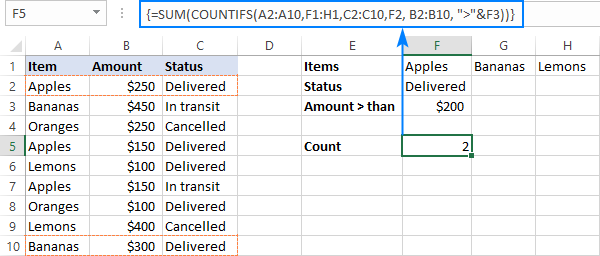## Count cells with multiple OR conditions

In the previous example, you have learned how to test one set of OR conditions. But what if you have two or more sets and you are looking to get a total of all possible OR relations?

Depending on how many conditions you need to handle, you can use either COUNTIFS with an array constant or SUMPRODUCT with ISNUMBER MATCH. The former is relatively easy to build, but it is limited to only 2 sets of OR conditions. The latter can evaluate any number of conditions (a reasonable number, of course, given Excel's limit to 255 arguments and 8192 characters to the total formula length), but it may take some effort to grasp the formula's logic.

### Count cells with 2 sets of OR conditions

When dealing with only two sets of OR criteria, just add one more array constant to the COUNTIFS formula discussed above.

For the formula to work, one minute but critical change is needed: use a horizontal array (elements separated by commas) for one criteria set and vertical array (elements separated by semicolons) for the other. This tells Excel to "pair" or "cross-calculate" the elements in the two arrays, and return a two-dimensional array of the results.

As an example, let's count "apples", "bananas" or "lemons" that are either "delivered" or "in transit":

`=SUM(COUNTIFS(A2:A10, {"apples", "bananas", "lemons"}, B2:B10, {"delivered"; "in transit"}))`

Please note the semicolon in the second array constant: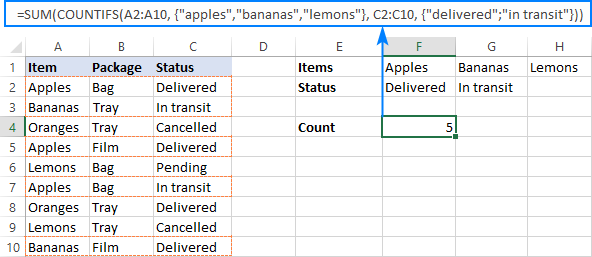Because Excel is a 2-dimentional program, it is not possible to construct a 3-dimentional or 4-dimentuional array, and therefore this formula only works for two sets of OR criteria. To count with more criteria, you will have to switch to a more complex SUMPRODUCT formula explained in the next example.

### Count cells with multiple sets of OR conditions

To count cells with more than two sets of OR criteria, use the SUMPRODUCT function together with ISNUMBER MATCH.

For example, let's get a count of "apples", "bananas" or "lemons" that are either "delivered" or "in transit" and are packaged in either "bag" or "tray":

```=SUMPRODUCT(ISNUMBER(MATCH(A2:A10,{"apples","bananas","lemons"},0))* ISNUMBER(MATCH(B2:B10,{"bag","tray"},0))* ISNUMBER(MATCH(C2:C10,{"delivered","in transit"},0))) ```

In the heart of the formula, the MATCH function checks the criteria by comparing each cell in the specified range with the corresponding array constant. If the match is found, it returns a relative position of the value if the array, N/A otherwise. ISNUMBER converts these values to TRUE and FALSE, which equate to 1 and 0, respectively. SUMPRODUCT takes it from there, and multiplies the arrays' elements. Because multiplying by zero gives zero, only the cells that have 1 in all the arrays survive and get summed.

Th screenshot below shows the result: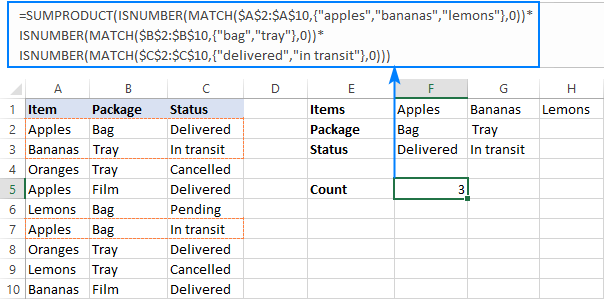This is how you use the COUNTIF and COUNTIFS functions in Excel to count cells with multiple AND as well as OR conditions. To have a closer look at the formulas discussed in this tutorial, you are welcome to download our sample Excel COUNTIF OR/AND workbook. I thank you for reading and hope to see you on our blog next week!